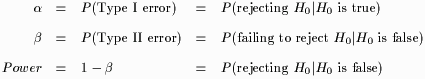## Power

This Web page accompanies the article:

Anderson-Cook, C. M., and Dorai-Raj, S. (2003), "Making the Concepts of Power and Sample Size Relevant and Accessible to Students in Introductory Statistics Courses using Applets," Journal of Statistics Education [Online], 11(3). (jse.amstat.org/v11n3/anderson-cook.html)

In hypothesis testing, Power is defined as the probability of rejecting the null hypothesis given that the null hypothesis is false.Figure 1. Probabilities in hypothesis testing.

Thus, power can be thought of as the rate at which signals are correctly identified (see Hypothesis Testing for more details).

In this section, six hypotheses (3 one-sample and 3 two-sample) are explored in terms of power:

1. Test on a binomial proportion:
The applets use the normal approximation (without continuity correction) to the binomial distribution. For the two-sided alternative, the hypotheses of interest for the one- and two-sample tests are given by Table 1.

 One-sample Two-sample H0 p = p0 p1 = p2 H1 pp0 p1p2
Table 1. Hypotheses for tests on proportions.

2. Test on a normal mean:
The applets allow varying assumptions for testing hypotheses on a normal mean. For the two-sided alternative, the hypotheses of interest for the one- and two-sample tests are given by Table 2.

 One-sample Two-sample H0=01 =2 H1012
Table 2. Hypotheses for tests on the mean.

The special cases are as follows:
1. Variance of the population(s) known.
In this case, the hypothesis is tested using the z-test in which the test statistic has a standard normal distribution (i.e. N(0,1)) under the null hypothesis.

2. Variance of the population(s) unknown.
In this case, the hypothesis is tested using the t-test. For the one-sample test, the distribution of the test statistic under the null hypothesis is tn-1 (i.e. t with n-1 degrees of freedom). For the two-sample test, the distribution of the test statistic under the null hypothesis is also t. However, the degrees of freedom are determined by whether the variances of each sample are assumed equal versus not equal. If the variances are assumed equal, the degrees of freedom are simply n1+n2-2. If the variances are assumed not equal, the degrees of freedom are determined by Satterthwaite's approximation (see the references or most statistics texts).

Problems:

1. The current standard for a particular manufacturer is that 80% of all products should be defect free. A quality control engineer claims that a newly designed process can improve the standard. The manufacturer will only invest in the new process if the yield is improved by at least 10%. Fifty products created under the new process are randomly selected and tested for defects. Assuming= 0.05, answer the following questions.

1. If in reality the new process improves the yield by 12%, what is the probability the new process will be still not be adopted?

2. How many more products should be tested so that the new process is accepted with a probability of at least 0.90?

2. Two species fish on average differ in length by 0.20cm. Scientists capture the 8 of each fish and measure their lengths. They estimate the standard deviation for each sample and record 0.25cm for the first species and 0.15cm for the second species. Assuming= 0.05 and unequal variances, answer the following questions:

1. What is the probability the scientists will detect the difference?

2. Assuming the scientists maintain equal sample sizes for each species, how many more fish should they capture to increase the probability of detecting the difference to 0.9?

3. If the scientists used= 0.01 instead, how would that change the previous two answers? Comment on the effects of balancingversus power.

Exact formulas for all power calculations can be found in the following references:

• Rosner, Bernard (2000) Fundamentals of Biostatistics, Duxbury, Pacific Grove, CA.
• Fleiss, Joseph (1981) Statistical Methods for Rates and Proportions, Wiley, New York.

View Instructions for this applet
 -- Select an applet -- Proportions Z-test T-test Open in new window   Open in current window